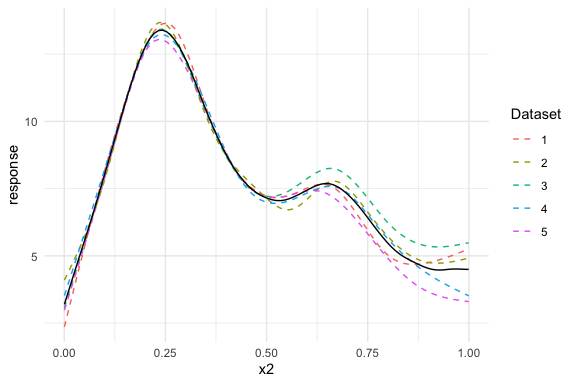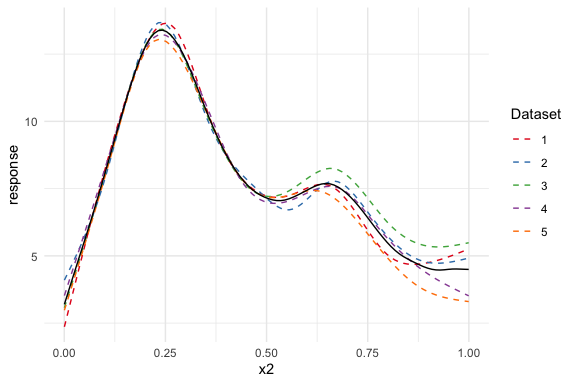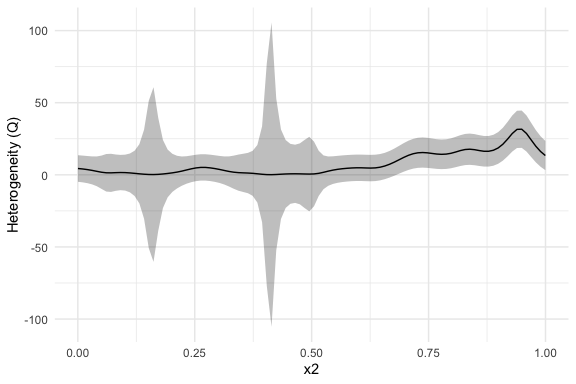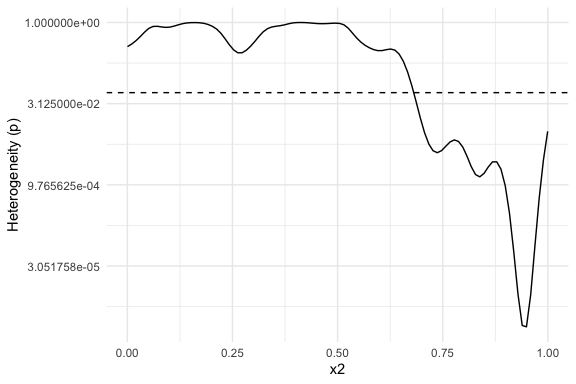# Heterogeneity Plots

The metagam package offers a way to visualize the heterogeneity of the estimated smooth functions over the range of explanatory variables. This will be illustrated here.

## Simulation

library("metagam")

We start by simulating 5 datasets using the gamSim() function from mgcv. We use the response $$y$$ and the explanatory variable $$x_{2}$$, but add an additional shift $$\beta x_{2}^{2}$$ where $$\beta_{2}$$ differs between datasets, yielding heterogeneous data.

library("mgcv")
#> This is mgcv 1.8-38. For overview type 'help("mgcv-package")'.
set.seed(1233)
shifts <- c(0, .5, 1, 0, -1)
datasets <- lapply(shifts, function(x) {
## Simulate data
dat <- gamSim(scale = .1, verbose = FALSE)
dat$y <- dat$y + x * dat\$x2^2
## Return data
dat
})

## Fit GAMs

Next, we analyze all datasets, and strip individual participant data.

models <- lapply(datasets, function(dat){
b <- gam(y ~ s(x2, bs = "cr"), data = dat)
strip_rawdata(b)
})

## Meta-Analysis

Next, we meta-analyze the models. Since we only have a single smooth term, we use type = "response" to get the response function. This is equivalent to using type = "iterms" and intercept = TRUE.

meta_analysis <- metagam(models, type = "response")

Next, we plot the separate estimates together with the meta-analytic fit. We clearly see that dataset 3, which had a positive shift $$\beta=1 x_{2}^2$$, lies above the others for $$x_{2}$$ close to 1, and opposite for dataset 5.

plot(meta_analysis)The plotting function for the meta-analysis object is a ggplot2-object, and can thus be altered using standard ggplot syntax. To learn more about customization of ggplot2-objects, please see the ggplot2 documentation.

plot(meta_analysis) +
ggplot2::scale_colour_brewer(palette = "Set1")We can investigate this further using a heterogeneity plot, which visualizes Cochran’s Q-test (Cochran (1954)) as a function of $$x_{2}$$. By default, the test statistic (Q), with 95 % confidence bands, is plotted. We can see that the confidence band for Q is above 0 for $$x_{2}$$ larger than about 0.7.

plot_heterogeneity(meta_analysis)We can also plot the $$p$$-value of Cochran’s Q-test. The dashed line shows the value $$0.05$$. The $$p$$-value plot is in full agreement with the Q-statistic plot above: There is evidence that the underlying functions from each dataset are different for values from about 0.7 and above.

plot_heterogeneity(meta_analysis, type = "p")